## Generalized Fibonacci Number

A generalization of the Fibonacci Numbers defined by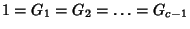and the Recurrence Relation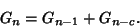(1)

These are the sums of elements on successive diagonals of a left-justified Pascal's Triangle beginning in the left-most column and moving in steps ofup and 1 right. The case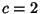equals the usual Fibonacci Number. These numbers satisfy the identities(2)(3)(4)(5)

(Bicknell-Johnson and Spears 1996). For the special case,(6)

Bicknell-Johnson and Spears (1996) give many further identities.

Horadam (1965) defined the generalized Fibonacci numbersas, where,,, andare Integers,,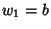, and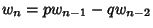for. They satisfy the identities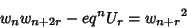(7)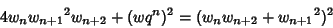(8)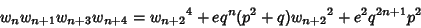(9)(10)
where(11)(12)

The final above result is due to Morgado (1987) and is called the Morgado Identity.

Another generalization of the Fibonacci numbers is denoted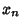. Givenand, define the generalized Fibonacci number byfor,(13)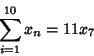(14)(15)

where the plus and minus signs alternate.

References

Bicknell, M. A Primer for the Fibonacci Numbers, Part VIII: Sequences of Sums from Pascal's Triangle.'' Fib. Quart. 9, 74-81, 1971.

Bicknell-Johnson, M. and Spears, C. P. Classes of Identities for the Generalized Fibonacci Numbersfor Matrices with Constant Valued Determinants.'' Fib. Quart. 34, 121-128, 1996.

Dujella, A. Generalized Fibonacci Numbers and the Problem of Diophantus.'' Fib. Quart. 34, 164-175, 1996.

Horadam, A. F. Generating Functions for Powers of a Certain Generalized Sequence of Numbers.'' Duke Math. J. 32, 437-446, 1965.

Horadam, A. F. Generalization of a Result of Morgado.'' Portugaliae Math. 44, 131-136, 1987.

Horadam, A. F. and Shannon, A. G. Generalization of Identities of Catalan and Others.'' Portugaliae Math. 44, 137-148, 1987.

Morgado, J. Note on Some Results of A. F. Horadam and A. G. Shannon Concerning a Catalan's Identity on Fibonacci Numbers.'' Portugaliae Math. 44, 243-252, 1987.